# NCERT Solutions For Class 8 Maths Chapter – 15 Introduction to graphs Exercise – 15.3

Q1. Draw the graphs for the following tables of values with suitable scales on  the axes.

• Cost of apples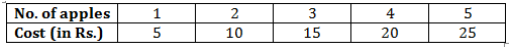• Distance travelled by a car.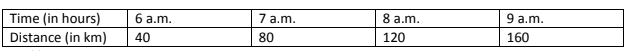(i)   How much distance did the car cover during the period 7:30 A.M.  to 8:00 A.M. ?

• What was the time when the car had covered a distance of 100 km since it’s start ?

( c ) Interest on deposits for a year.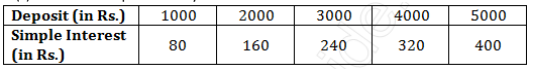8 chapter 15-16" />

• Does the graph pass through the origin ?
• Use the graph to find the interest on Rs 2500 for a year.
• To get an interested of Rs 280  per year, how much money should be deposited ?

Solution:-

Mark “ number of apples” on x- axis and “cost” on y – axis . The graph is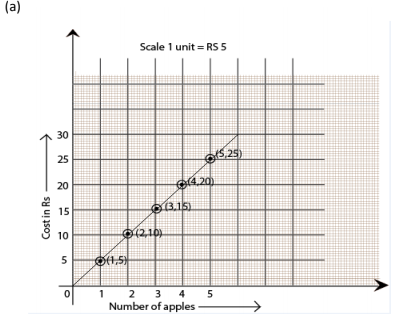( b ) Represent the “time” on x – axis and “distance on y- axis.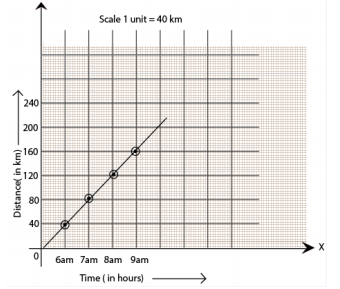• The car covered 20 km distance
• It was 7:30 A.M. when it covered 100 km distance.
• Represent “Deposit” on y – axis and simple “interest” on x- axis.

( c ) Represent “Deposit” on y- axis and “simple interest” on x -axis.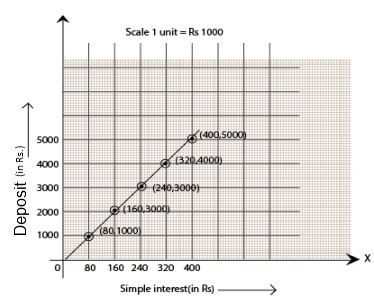• Yes, the graph passes through the origin.
• Interest on Rs. 2500 is Rs. 200 for a year.
• 3500 should be deposited for interest of Rs. 280.

NCERT Solution for class 7th English Poem-8

Q2. Draw a graph for the following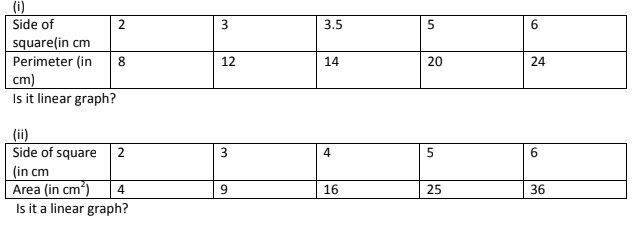Solution:-

• Yes, it is linear graph.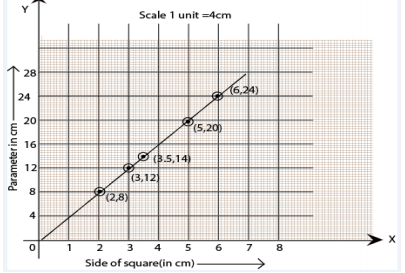• No, it is not a linear graph because the graph does not provide a straight line.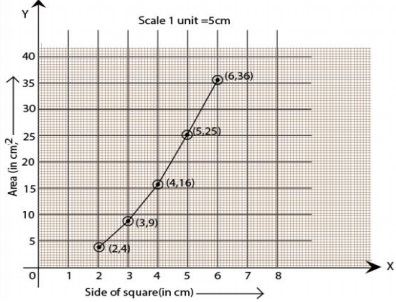NCERT Solutions For Class 8 Science Chapter – 2 Microorganisms: Friend and Foe

NCERT Solutions For Class 8 Maths Chapter – 15 Introduction to graphs Exercise – 15.3 Q1. Draw the graphs for the…